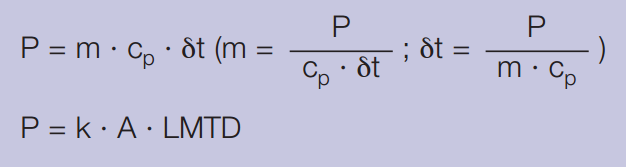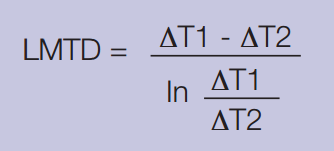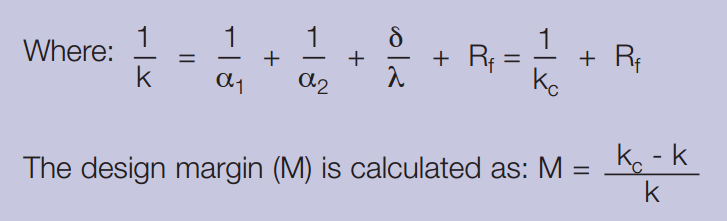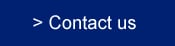To solve a thermal problem, we need to know several parameters. Further data can then be determined.

The six most important parameters include:

• The amount of heat to be transferred (heat load)
• The inlet and outlet temperatures on the primary and secondary sides
• The maximum allowable pressure drop on the primary and secondary sides
• The maximum operating temperature
• The maximum operating pressure
• The flowrate on the primary and secondary sides

If the flow rate, specific heat and temperature difference on one side are known, the heat load can be calculated.

Calculation method

The heat load of a heat exchanger can be derived from the following two formulas:

1. Heat load, Theta and LMTD calculationWhere:

m = mass flow rate (lb/h)

cp = specific heat (btu/lb °F)

δt = temperature difference between inlet and outlet on one side (°F)

k = heat transfer coefficient (btu/ft2 h °F)

A = heat transfer area (ft2)

LMTD = log mean temperature differenceT1 = Inlet temperature - hot side

T2 = Outlet temperature - hot side

T3 = Inlet temperature - cold side

T4 = Outlet temperature - cold side

LMTD can be calculated by using the following formula, where ∆T1 = T1–T4 and ∆T2 = T2–T32. Heat transfer coefficient and design margin

The total overall heat transfer coefficient k is defined as:α1 = The heat transfer coefficient between the warm medium and the heat transfer surface (btu/ft2 h °F)

α2 = The heat transfer coefficient between the heat transfer surface and the cold medium (btu/ft2 h °F)

δ = The thickness of the heat transfer surface (ft)

Rf = The fouling factor (ft2 h °F/btu)

λ = The thermal conductivity of the material separating the medias (btu/ft h °F)

kc = Clean heat transfer coefficient (Rf=0) (btu/ft2 h °F)

k = Design heat transfer coefficient (btu/ft2 h °F)

M = Design Margin (%)

Combination of these two formulas gives: M = kc · Rf

i.e the higher kc value, the lower Rf-value to achieve the same design margin.

For a more complete explanation of heat transfer theory and calculations, download the following brochure:

The theory behind heat transfer

How GPHEs work

Selection guide

Features that matter

Plate technology

GPHE vs shell-and-tube

Calculation method

Types of GPHEs

Servicing a GPHE